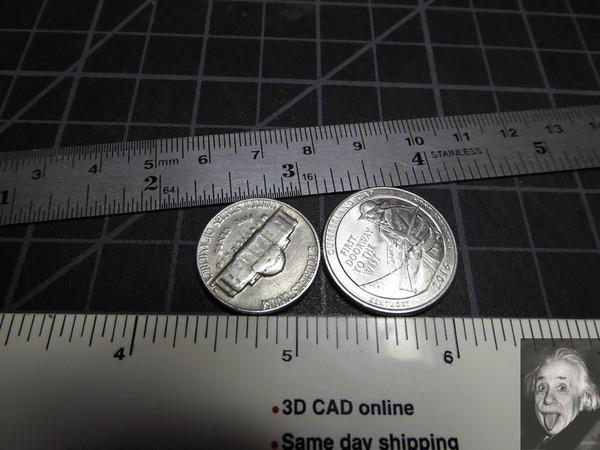Free 173 Best Homemade Tools eBook:

# Thread: Accurate angles without a sinebar

1. ## Accurate angles without a sinebar

Novice metalworkers may not yet have a sinebar yet may encounter a situation where they need to machine an angle accurately. Folks who use angle blocks in lieu of a sinebar may not have the blocks needed to produce a particular angle.

All is not lost. The one accurate tool most budding machinists have available is a lathe. Using only this tool, it's possible to manufacture a precise quasi-sinebar for any angle of interest. The procedure depends on the following statement:

If I take two cylinders of diameters d1 (smaller) and d2 (larger) and lay them in contact with each other on a horizontal plate, a flat plate placed across them will form an angle, theta, with the horizontal given by:

d1/d2 = (1-sin(theta/2))/(1+sin(theta/2))

To visualize this (and because every tool post needs a picture), look at this cartoon of the process...The white plastic scale represents the horizontal surface, e.g., the floor of the mill vise opening. The coins represent the two cylinders resting on the horizontal and contacting each other. The metal scale represents the platform for the part and makes an angle of theta with the plastic scale.

So, how does this work?

Given that you need the angle theta, plug that value into the right side of the equation and compute the ratio of d1/d2. [A scientific calculator will be a big help. They're available cheaply and essential in any shop with pretenses of accuracy.] This ratio will always have a value less than one.

Select a piece of stock of suitable size for d2 and measure its true diameter. Multiply the latter by the ratio calculated above to obtain the value of d1. Use the lathe to turn down a chunk of d2 to a diameter of d1. Simple and straightforward.

I'll run through an example to illustrate the calculation process.

Let's say I need an angle of 3.6 degrees. Then

theta = 3.6
theta/2 = 1.8

1 - sin(theta/2) = 1 - 0.0314 = 0.9686
1 + sin(theta/2) = 1 + 0.0314 = 1.0314

d1/d2 = 0.9896 / 1.0314 = 0.9391

Let's say I choose to use a piece of accurate 0.5" drill rod as d2. Then

d1 = 0.9391 * 0.5 = 0.4695"

So I machine the drill rod down to 0.4695" and put that chunk next to a chunk of the 0.5" material and, voila, I have my angle.Reply With Quote

2. ## The Following 2 Users Say Thank You to mklotz For This Useful Post:

benkeller3 (Jan 18, 2017), PJs (Jan 19, 2017)

3. Marv,

I hate to be a damper on this but I believe that you made an error in your calculations. Sin(1.8) is not equal to 0.0134, it is over twice that at 0.0314. I think that maybe you transposed the 31 to 13. So using the correct value you get a d1=0.4695 not 0.4883".
I love these little maths tricks but I always like to do an error analysis so that you have a good idea how accurate you need to be. For example in your case with d2=0.5" and a desired angle of 3.6 deg then an error of 1 thou on either diameter will give an error of approx. 0.1 deg. Going for larger diameters reduces that sensitivity. e.g if d2=1" then it would take near 2 thou error on diameter to get the same angular error.
The sensitivity to error will vary with the required angle so it pays to check error sensitivity for the angle that you need.
Another thing to be aware of is that different calculators use different algorithms for trig. functions and so give slightly different answers. e.g. My TI calculator (and the Windows 10) both give sin(45) as 0.707106781 but I know that the correct value is 0.7070707070707 etc. not a huge difference but important enough when working to tenths.
You may wonder how I spotted the incorrect sin value. I didn't reach for a calculator to find errors but used a couple of mental maths tricks and the error just shouted out. Firstly, I know that the sin (and tan) of a small angle (say less than 10deg) is close to being equal to the angle itself expressed in radians. Secondly, I know that there are very close to 57.3 deg. in a radian. So mentally I approximated the 1.8 deg. to 2 and radians to 60 which gave me the sin as 2/60=1/30=0.0333, so I knew immediately that 0.0134 was not accurate. This often known as the "small angle approximation". Then a calculator confirmed the value to be 0.0314 which is close to my estimate.Reply With Quote

4. You're absolutely correct, Tony. I transposed the numbers and didn't bother to check my work. Thanks for the correction; I've fixed the errors in the original post.Reply With Quote

5. ## The Following 3 Users Say Thank You to mklotz For This Useful Post:

Christophe Mineau (Jan 15, 2017), Paul Jones (Jan 15, 2017), PJs (Jan 19, 2017)

6. Thanks Marv, cristal clear explanationReply With Quote

7. Thanks mklotz! We've added your Angle Finding Method to our Measuring and Marking category,
as well as to your builder page: mklotz's Homemade Tools. Your receipt:Reply With Quote

#### Thread Information

##### Users Browsing this Thread

There are currently 1 users browsing this thread. (0 members and 1 guests)

####Posting Permissions

• You may not post new threads
• You may not post replies
• You may not post attachments
• You may not edit your posts
•